# Simplifying Ratios

Here we will learn about simplifying ratios, including how to simplify a ratio, how to write equivalent ratios and using simplifying ratio in real life problems.

There are also ratio worksheets and word problems based on Edexcel, AQA and OCR exam questions, along with further guidance on where to go next if you’re still stuck.

## What is simplifying ratios?

Simplifying ratios is a way of using common factors to divide all the numbers in a ratio until they cannot be divided further.

A simplified ratio is equivalent to an non simplified ratio.

E.g.

Let’s look at 12 red counters and 16 blue counters.

If we organised them into a the two colours, we can get something that looks like this:

Each of the four rows contains 3 red counters and 4 blue counters. This allows us to express the ratio 12:16 as the ratio in the simplest form 3:4 .

We can simplify the ratio by finding the highest common factor or HCF (otherwise known as the greatest common factor or GCF) of each of the numbers in the ratio.

For the example above, the highest common factor would be 4 as we can divide 12 and 16 by 4 .

### Bar modelling

We can use bar modelling to help us simplify ratios.

E.g.

Here are some circles.

Rearranging them into coloured bars, we can show the number of orange circles and the number of purple circles.

The number of orange circles is 4 , and the number of purple circles is 6 . This can be written as the ratio 4:6.

We can also rearrange these circles into another pattern:

Here, we still have the ratio of 4:6 but we have arranged them into two rows, each with the same proportion of circles.

Each row contains 2 orange circles, and three purple circles. This means that we can divide each side of the ratio by 2 , and still obtain the same ratio of orange to purple.

So by using bar modelling, we can simplify the ratio 4:6 to get 2:3.

### How to simplify a ratio using the HCF

The fastest way to simplify a ratio is to use the highest common factor of the numbers in the ratio.

For example

If you are given a ratio of 64:80, to simplify it: first find the highest common factor which is 16; next divide each part of the ratio by the highest common factor 64 \div 16 : 80 \div 16 which gives you the answer 4:5

### Ratio Simplifier

We can summarise simplifying ratios using this formula

The simplified ratio \mathrm{A}: \mathrm{B}=A \div H C F \text{ of }(A, B): B \div H C F \text{ of }(A, B)

For example.

Simplify 45:75

HCF of (45,75) = 15

\begin{aligned} 45: 75 & =45 \div 15: 75 \div 15 \\\\ & =3: 5 \end{aligned}

### What is simplifying ratios?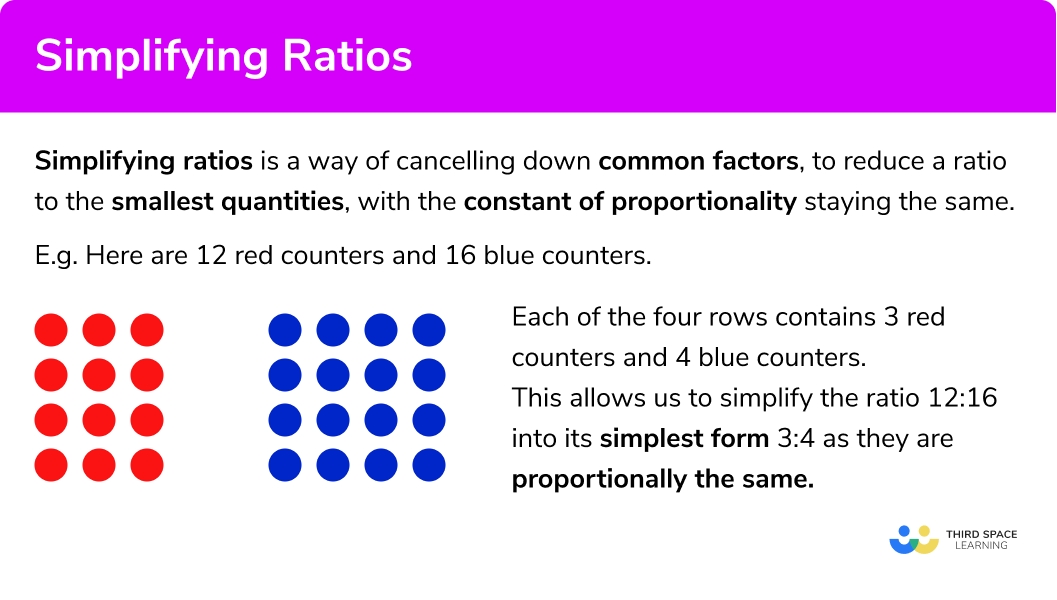## How to simplify a ratio

In order to simplify a ratio:

1. Calculate the highest common factor of the parts of the ratio.
2. Divide each part of the ratio by the highest common factor.

### How to simplify a ratio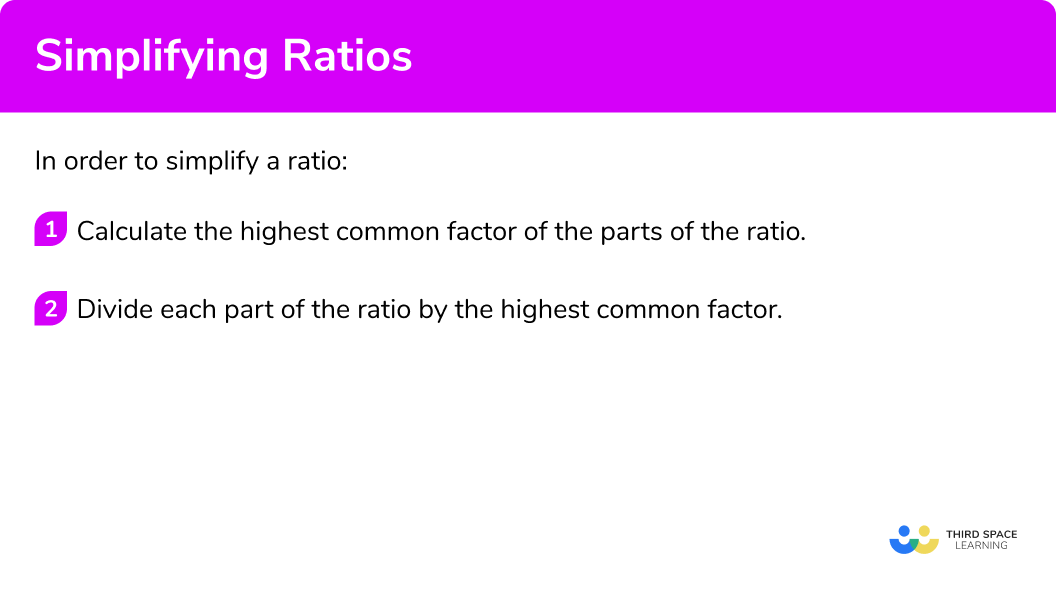## Related lessons on ratio

Simplifying ratios is part of our series of lessons to support revision on ratio. You may find it helpful to start with the main ratio lesson for a summary of what to expect, or use the step by step guides below for further detail on individual topics. Other lessons in this series include:

## Simplifying ratios examples

### Example 1: standard question

Simplify the ratio

20:32

1. Calculate the highest common factor of the parts of the ratio.

Listing the factors of 20 and 32 , we have:

20 = 1, 2, 4, 5, 10, 20

32 = 1, 2, 4, 8, 16, 32

HCF(20,32) = 4

2Divide each part of the ratio by the highest common factor.

Solution:

5:8

### Example 2: three part ratio

Simplify the following ratio

21:14:28

Listing the factors of all 3 parts of the ratio, we have

21 = 1, 3, 7, 21

14 = 1, 2, 7, 14

28 = 1, 2, 4, 7, 14, 28

HCF(21,14, 28) = 7

Solution:

3:2:4

### Example 3: ratio including decimals

Simplify the following ratio

1.6:2.4

We currently have two decimal values within the ratio. As we only find factors of whole numbers, we need to determine an equivalent ratio first. By multiplying both sides by 10 , we obtain the ratio 16:24 which we can now find common factors of.

Listing the factors of each part of the ratio, we have

16 = 1, 2, 4, 8, 16

24 = 1, 2, 3, 4, 6, 8, 12, 24

HCF(16, 24) = 8

Solution:

2:3

### Example 4: fractions

Simplify the ratio

\frac{1}{2}:\frac{1}{6}

Here we have two fractions. Instead of looking at finding the highest common factor of fractions, we need to determine an equivalent ratio that contains whole numbers. We can do this by multiplying both fractions by a number (preferably their lowest common multiple) to give a ratio with integers:

\frac{1}{2}\times 12 = 6

\frac{1}{6}\times 12 = 2

We can now state that the ratio \frac{1}{2}:\frac{1}{6} is equivalent to 6:2 . (Be careful of the order) and HCF(6,2) = 2.

Solution:

3:1

### Example 5: mixed numbers

Simplify the ratio

1\frac{1}{4}:2\frac{2}{5}

As we have two mixed numbers, we can convert them to improper fractions or decimals. We will look at converting the mixed numbers to decimals and then simplify the ratio.

Converting both of the mixed numbers to decimals we have 1\frac{1}{4}:2\frac{2}{5} s the same as 1.25:2.4

Multiplying both of these parts of the ratio by 100 will quickly remove the decimal so we can then simplify the ratio.

125:240

Calculating the highest common factor of 125 and 240 quickly by using prime factor trees, we get:

125 = 5\times 5\times 5

240 = 2\times 2\times 2\times 2\times 3\times 5

From this, we can show that the highest common factor of 125 and 240 is 5.

HCF(125,240) = 5

Solution:

25:48

### Example 6: algebraic terms

Given that a, b, and c are all positive integers, simplify the ratio

ab^{2}:bc

ab^{2}=a\times b\times b

bc=b\times c

HCF(ab^{2},bc) = b

Solution:

ab:c

### Example 7: bar modelling

Use a bar model to simplify the ratio 8:10

Representing the ratio 8:10 as a bar model, we have

Where red represents 8 shares, and purple represents 10 shares.

HCF(8,10)=2

As the highest common factor is 2 , we divide the bar above into two bars containing the same amount of red shares, and purple shares.

Each bar is the ratio 4:5.

Solution:

4:5

### Common misconceptions

• Not fully simplified

A common error is that the ratio is not cancelled to its simplest form due to not using the highest common factor,

E.g.

Simplify the ratio 12:18.

We can simplify by dividing parts of the ratio by dividing both numbers by 2 , leaving the ratio 6:9 .

This is not the final answer as it can be simplified further by dividing by 3 to get the ratio 2:3.

• Solution not written in the correct order

A common error is to change the order of the ratio. It is important that the order of the numbers in the ratio remains the same while simplifying.

• Not simplifying all parts of the ratio

When simplifying a ratio with more than two parts, a common misconception is to not simplify all of the parts of the ratio.

E.g.

“Simplify the ratio 12:6:15 ” could be incorrectly written as 2:6:3 as the middle part is not cancelled down.

• Incorrect division

Some numbers are more tricky to divide than others, especially when calculating it mentally.

Common incorrect calculations include 15\div 5=5, 32\times 7=4, and 24\div 8=4

We cannot use addition and subtraction to simplify a ratio, we can only use multiplication and division.

Let’s look at why using a visual representation:

Below are 15 rectangles (r) and 5 stars (s) .

If we were to express the number of stars and rectangles as a ratio, we would write the ratio 5:15 . Remember that the order is important and so we have written the number of stars first and the number of rectangles second (s:r) .

Dividing the number of rectangles by the number of stars we get \frac{r}{s}=3 .

Here the number 3 is known as the constant of proportionality (Step-by-step guide: Direct proportion).

Now if we were to subtract 1 from each of the stars and the rectangles above and divide the quantities again the answer should be 3 if the ratio was equivalent.

Subtracting 1 from each we now have 4 stars (S) and 14 rectangles (R) . I \frac{R}{S}=\frac{14}{4}=3.5 .

As this is not 3 the ratios are not equivalent.

• Ratios and decimals

Simplified ratios should not include decimals.

E.g.

If we want to simplify the ratio 9:12 , we need to divide each quantity in the ratio by a common factor.

Here we have simplified by a common factor of 3 , and then simplified again by dividing these new numbers by 4 .

When simplifying a ratio, your solution must contain whole numbers (integers)

So the correct simplified ratio is:

### Practice simplifying ratios questions

1. Simplify

36:30

6:55:612:1013:15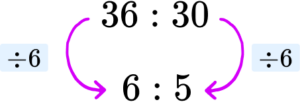2. Simplify

12:18:30

6:3:106:9:154:9:102:3:5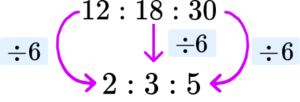3. Simplify

0.8:1.6

8:162:11:24:8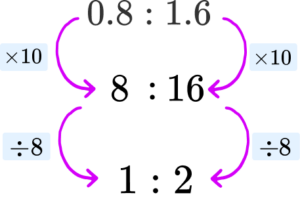4. Simplify

\frac{3}{4}:\frac{4}{5}

4:53:415:1675:80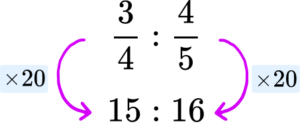5. Simplify

3\frac{1}{2}:1\frac{3}{4}

3:1350:1753.5:1.752:1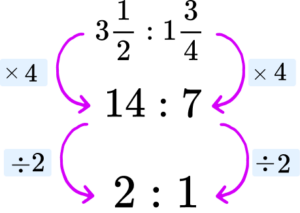6. Given that x and y are positive integers, simplify

2x^{3}:x^{2}y

2:12x:y2:y2x:xy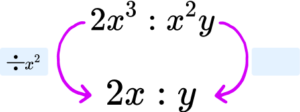### Simplifying ratios GCSE questions

1. The number of visitors to a zoo is written as the ratio of adults to children as 252:306 respectively. Write this ratio in its simplest form.

(1 mark)

14:17

(1)

2. (a) A taxi firm is researching the distances travelled for each journey. The results are shown below.State the ratio of journeys between 5 and 10 miles and the total number of journeys. Write your answer in its simplest form.

(b) Another 14 journeys are added to the table, 6 of which are within the category 5\leq m<10 . What is the new ratio of the number of journeys between 5 and 10 miles and the total number of journeys. Write your answer in its simplest form.

(3 marks)

(a)

42:114

(1)

(b)

New ratio 48:128

(1)

3:8

(1)

3. Below is a recipe to make custard for 8 people.(a) Write the ratio of caster sugar to double cream to milk in its simplest form.

(b) I only have 3 egg yolks. How much double cream should I use?

(4 marks)

(a)

100g:200ml:700ml

(1)

1:2:7

(1)

(b)

4:200=1:50=3:150

(1)

150ml double cream

(1)

## Learning checklist

You have now learned how to:

• Use ratio notation, including reduction to simplest form

## Still stuck?

Prepare your KS4 students for maths GCSEs success with Third Space Learning. Weekly online one to one GCSE maths revision lessons delivered by expert maths tutors.

Find out more about our GCSE maths tuition programme.